# Basic Course on Turbulence and Turbulent Flow Modeling 16: 16.1 Analysis overview, 16.2 Analysis results

#### 16.1: Analysis overview

In this column, we will see the results of a flow analysis around a golf ball as a simulation of a familiar phenomenon. Golf balls are very interesting objects fluid-dynamically. Do you know why the surface of a golf ball is not smooth but rather there are a lot of dents? The reason is said to be that those dents called dimples reduce air drag on a golf ball. Such a minuscule difference of the surface helps reduce air drag, and as a result, helps improve the driving distance greatly. Let us analyze the intriguing flow around a golf ball. We take two types of golf ball, one with dimples and one without, as shown in Figure 16.1, and focus on the effect of the dimples.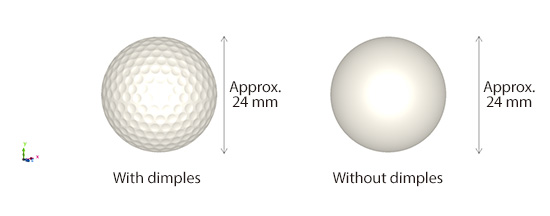Figure 16.1: Golf ball models

The analysis is performed with a model shown in Figure 16.2, where a golf ball is placed inside a rectangular box of air. Although golf balls fly through the air in reality, the ball is fixed in space and the air is blown in at the flying speed of the golf ball. In this analysis, the flying speed of 300 km/h is assumed. This setup enables an analysis of a golf ball in flight. Note, however, that the effect of the rotation of the golf ball is not taken into account. The calculations are performed with LES.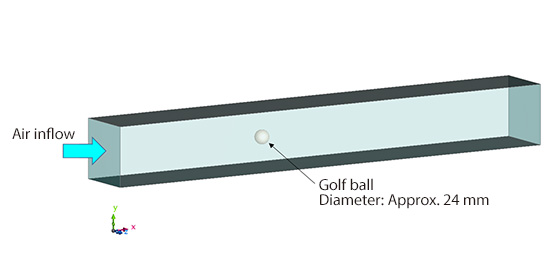Figure 16.2: Model for a flow analysis around a golf ball

#### 16.2: Analysis results

Let us see the results of flow analysis around a golf ball. Figure 16.3 is the flow field around the golf ball. In the top figure for the case with dimples, we can see small vortices generated from the top and bottom of the golf ball. On the other hand, for the case without dimples, there is a large vortex behind the golf ball. How vortices are generated behind the ball differs greatly between the cases with and without dimples.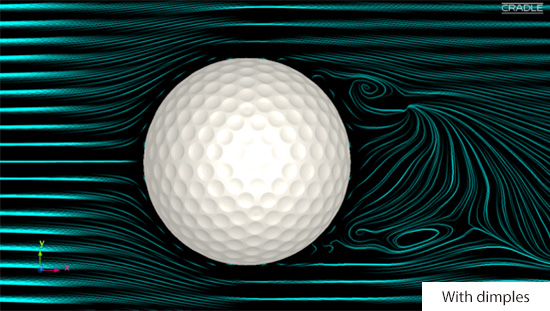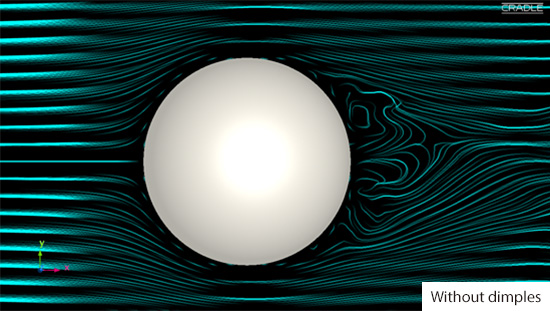Figure 16.3: Flow field around the golf ball

How did this difference in vortex structure arise? Let us take a closer look at the flow field near the surface of the golf ball. Figure 16.4 shows a magnified view of the flow field near the golf ball. For the case with dimples, we can see small eddies in each dimple. These eddies interact with each other to form a complex flow toward downstream. In contrast, for the case without dimples, there are no eddies generated on the surface, and a large vortex is generated when the flow separates from the surface behind the ball.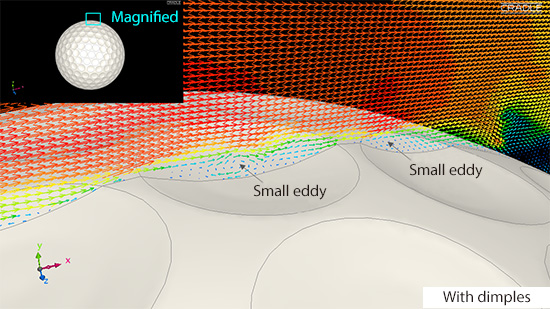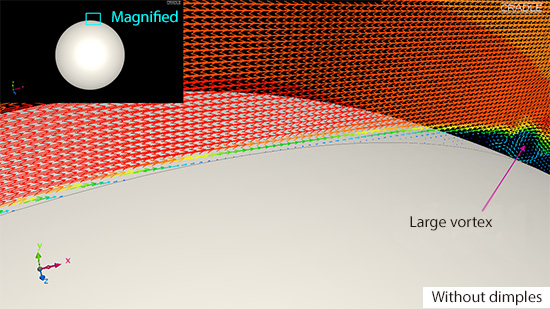Figure 16.4: Flow field near the surface of the golf ball

Next, let us see the vortex tubes around the golf ball. For the case with dimples, we can see a lot of fine vortex tubes generated at the surface dimples just as we saw in the cross-sectional vector plot in Figure 16.4. For the case without dimples, there is no vortex tube generated on the surface of the ball.

In this column, we have seen the generation of many small eddies near the surface of the golf ball thanks to the dimples. How does this generation of small vortices affect the reduction of air drag? We will discuss that in the next column.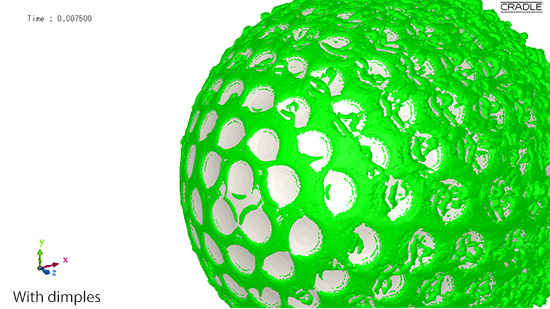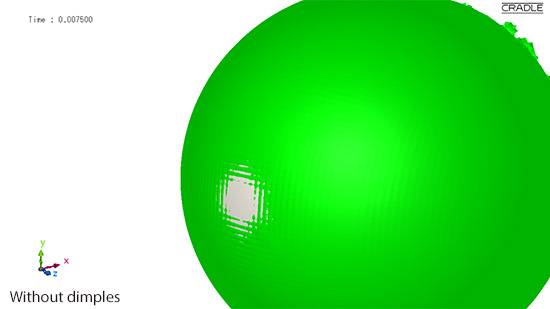Figure 16.5: Vortex tubes near the surface of the golf ball